# Python Learning Week 5 (Solution)

Write a program in python to implement given Graph, BFS and DFS.  Output must be as given below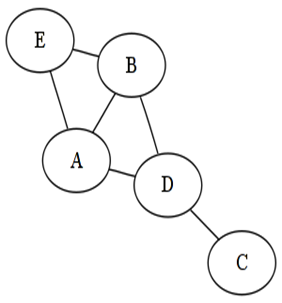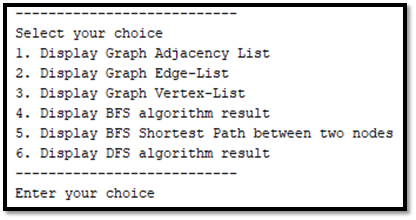CODE:

graph {

‘E’ [‘A’,‘B’],

‘A’ [‘D’],

‘B’ [‘D’],

‘D’ [‘C’],

‘C’:   []

}
#EDGE-LIST
def edges(graph):

edge_list []

for node in graph:
for
neighbour in graph[node]:

edge_list.append((nodeneighbour))

return edge_list

#VERTEX-LIST
class Vertices_list:
def
__init__(self,graph_dict=None):
if
graph_dict is None:

graph_dict []

self.graph_dict graph_dict

def get_Vertices(self):
return
list(self.graph_dict.keys())
Vertices_list(graph)

#BFS ALGORITHM
import collections
def bfs(graphroot):

visitedqueue set(), collections.deque([root])

while queue:

vertex queue.popleft()

print(str(vertex” “end=“”)

for neighbour in graph[vertex]:
if
neighbour not in visited:

queue.append(neighbour)

#SHORTEST-PATH

def shortest_path(graphstartendpath=[]):

path path [start]

if start == end:
return
path

shortest = None
for
node in graph[start]:
if
node not in path:

newpath shortest_path(graphnodeendpath)

if newpath:
if not
shortest or len(newpathlen(shortest):

shortest newpath

return shortest

#DFS ALGORITHM

def dfs(graphnodevisited):
if
node not in visited:

visited.append(node)

for in graph[node]:

dfs(graph,kvisited)

return visited

2. Display Graph Edge-List
3. Display Graph Vertex-List
4. Display BFS Algorithm Result
5. Display BFS Shortest Path between two nodes
6. Display DFS  Algorithm Result
\n”’))

if == 1:

print(graph
elif == 2:

print(‘Graph Edge List ‘)

print(edges(graph))
elif == 3:

print(‘Graph Vertex List ‘)

print(G.get_Vertices())
elif == 4:

print(‘BFS Algorithm Result’)

bfs(graph‘E’)
elif == 5:

first input(“Enter FIRST node “)

second input(“Enter SECOND node “)

print(‘Shortest Path between two nodes is :’)

print(shortest_path(graphfirstsecond))
elif == 6:

print(‘DFS  Algorithm Result’)

visited dfs(graph‘E’, [])

print(visited)

else:

print(“wrong option”)

OUTPUT: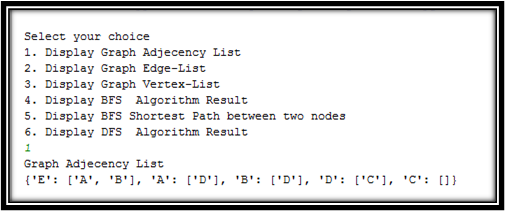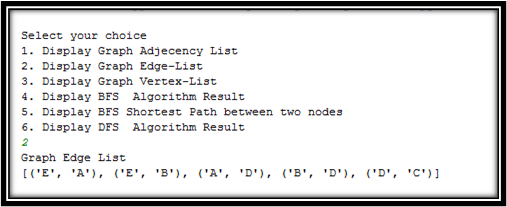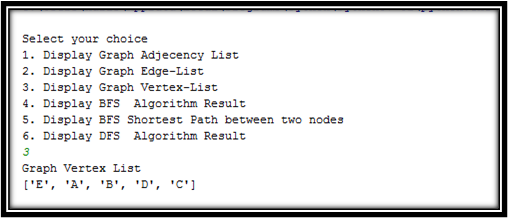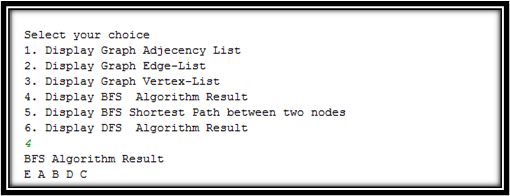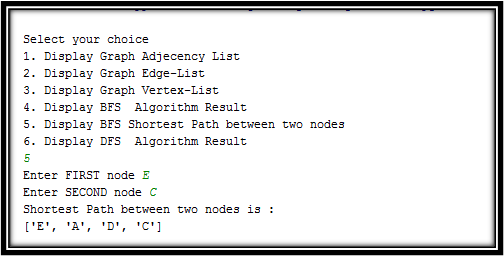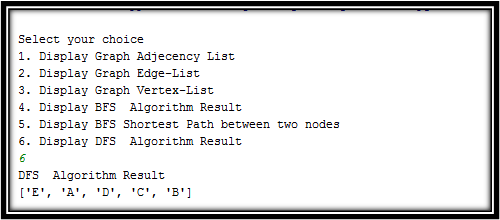Implement given graph in python. Traverse Graph using DFS traversals. The starting node is ‘Frankfurt’ and goal node is ‘Munchen’.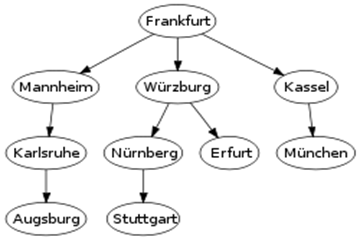CODE:

graph {

‘Frankfurt’[‘Mannheim’‘Wurzburg’‘Kassel’],

‘Mannheim’[‘Karlsruhe’],

‘Karlsruhe’[‘Augsburg’],

‘Wurzburg’[‘Nurnberg’‘Erfurt’],

‘Nurnberg’[‘Stuttgart’],

‘Kassel’[‘Munchen’],

‘Augsburg’[],

‘Stuttgart’[],

‘Erfurt’[],

‘Munchen’[]

}
def dfs(graphnodevisited):
if
node not in visited:

visited.append(node)

for in graph[node]:

dfs(graph,kvisited)

return visited

print(‘Traversing graph from “Frankfurt” to “Munchen”‘)
visited dfs(graph‘Frankfurt’, [])
print(visited)

OUTPUT: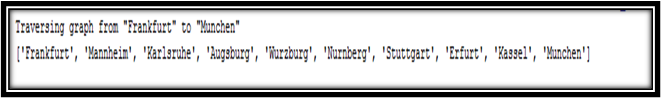What we studied in Week 5:

In this week we study Uninformed Search  that is Depth First Search (DFS) and Breadth First Search (BFS). Basically DFS & BFS  is an algorithm for traversing , searching tree and graph data structures. DFS algorithm starts at the root node and explores as far as possible along each branch before backtracking. In BFS starts at the root node explores all of the neighbor nodes at the present depth prior to moving on to the nodes at the next depth level. DFS will process the vertices first deep and then wide after processing a vertex its recursively processes all of its descendants. DFS uses stack (LIFO) data structure and BFS uses Queue (FIFO) data structure and we implemented both searches in python. This is all we have studied in this week.